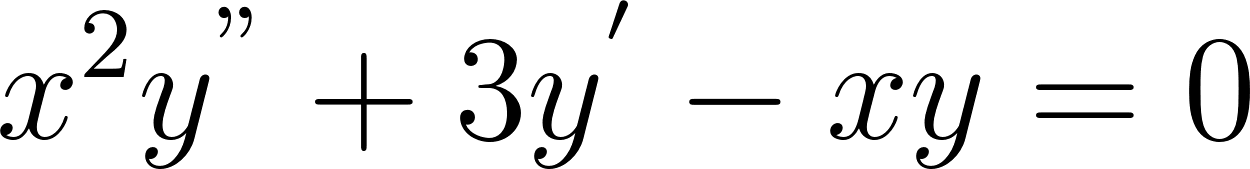×
Get Full Access to Fundamentals Of Differential Equations - 8 Edition - Chapter 8.3 - Problem 2e
Get Full Access to Fundamentals Of Differential Equations - 8 Edition - Chapter 8.3 - Problem 2e

×

# In 1–10, determine all the singular points of | Ch 8.3 - 2EISBN: 9780321747730 43

## Solution for problem 2E Chapter 8.3

Fundamentals of Differential Equations | 8th Edition

• Textbook Solutions
• 2901 Step-by-step solutions solved by professors and subject experts
• Get 24/7 help from StudySoup virtual teaching assistantsFundamentals of Differential Equations | 8th Edition

4 5 1 387 Reviews
12
4
Problem 2E

In Problems 1–10, determine all the singular points of the given differential equation.

$$x^{2} y^{\prime \prime}+3 y^{\prime}-x y=0$$

Equation Transcription:Text Transcription:

x2y''+3y'-xy=0

Step-by-Step Solution:

Step 1 of 3

Given the differential equationStep 2 of 3

Step 3 of 3

## Discover and learn what students are asking

Unlock Textbook Solution